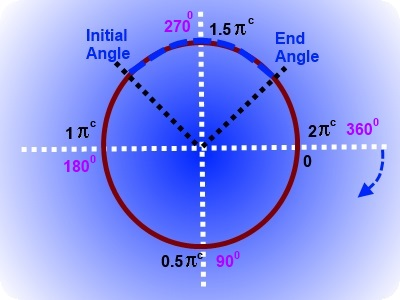### Drawing Curves

In this tutorial, you will learn how to draw curves; they include the following:

• How to draw an arc.
• How to turn an arc into a circle.
• How to draw a quadratic curve.
• How to draw a Bezier curve.
• Interactive programmes to practise the role of centre and end points.
Finding a good book to master HTML5 can be very challenging: there are so many around - most with eye-catching titles and very complex substance.
Therefore, Vivax Solutions strongly recommends Core HTML5 Canvas for those who really want to delve into HTML5.
Please click the image to access Amazon:

Arc

In order to draw an arc, we must specify a centre, a radius, a start angle, an end angle and the direction in terms of of a Boolean value - clockwise/anticlockwise.

The angles must be given in radians.

If you are not familiar with radians, you can easily convert degrees into radians by multiplying the value in degree by π/180.

It is very important to get a clear sense of the convention of measuring the angles. The following diagram explains this:30 * π /180
= 30 * π / 180
= π/6
= 0.5235c

E.g.

context.arc(200, 150, 80, 1.25π, 1.75π, false) draws an arc of 80 pixels, as follows:

You can change its shape by giving the initial angle and final angle in degrees - 00 to 3600 - in the following text boxes:

Ini_Angle:   End_Angle:

The Code for an Arc is as follows:

<canvas id="Canvas_Four" width="400" height="300" style="border:5px solid red;"></canvas>
<script type="text/javascript">
var canvas = document.getElementById('Canvas_Four');
var cnt = canvas.getContext('2d');
// Draw an arc
cnt.beginPath(); cnt.arc(200, 150, 80, 1.25*Math.PI, 1.75*Math.PI, false);
cnt.lineWidth = 8;
cnt.strokeStyle = "blue";
cnt.stroke();
</script>

A quadratic curve, also known as a parabola in mathematics, can be drawn on canvas by context.quadraticCurveTo() method.

First of all, context.moveTo() method must be used to specify the beginning of the curve, also known as the context point. The two arguments of context.quadraticCurveTo() method are the pair of coordinates of the centre point and end point respectively.

First, read the code; then, click the interactive button to see the effect on the curve on the canvas, when the centre point is changed.

Coordinates of the Centre Point:

The Code for Quadratic Curve is as follows:

<canvas id="Canvas_Five" width="400" height="300" style="border:5px solid red;"></canvas>
<script type="text/javascript">
var canvas = document.getElementById('Canvas_Five');
var cnt = canvas.getContext('2d');
cnt.beginPath();
// beginning of the curve - context point
cnt.moveTo(100, 250);
// coordinates of the centre point and end point
cnt.lineWidth = 10;
// line color
cnt.strokeStyle = 'green';
cnt.stroke();

Bezier Curve

A Bezier curve can be drawn on canvas by context.bezierCurveTo() method.

First of all, context.moveTo() method must be used to specify the beginning of the curve, also known as the context point. The three arguments of context.bezierCurveTo() method are the sets of coordinates of two control points and the end point respectively.

First, read the code; then, click the interactive button to see the effect on the curve on the canvas, when the control points are changed.

The Code for Bezier Curve is as follows:

<canvas id="Canvas_Five" width="400" height="300" style="border:5px solid red;"></canvas>
<script type="text/javascript">
var canvas = document.getElementById('Canvas_Five');
var cnt = canvas.getContext('2d');
cnt.beginPath();
// beginning of the curve - context point
cnt.moveTo(100, 250);
// coordinates of the centre point and end point
cnt.lineWidth = 10;
// line color
cnt.strokeStyle = 'green';
cnt.stroke();

Amazon Best SellerEverything is evolving; so is the layout of a text book. By uniquely presenting the rich contents of the book, the author has elevated positive user experience of reading a text book to a new level: the examples are easy to follow and rich in standard. Highly recommended for those who want to master JavaScript and JQuery.

### Progressive Web Apps(PWA)The significance of app stores is over; progressive web apps is the next big thing. They are just websites that makes the need of going through app stores and need of storing redundant. They work offline too. If you have a reasonable understanding of HTML, CSS and JavaScript, this is the book for you to learn in no time.

### HTML5 Canvas AnimationsDavid Geary, in this book, shows how to combine JavaScript and HTML5 Canvas to produce amazing animations; he has set aside a whole chapter to teach you the role of physics in animations. If you want an in-depth understanding about HTML5 Canvas animations, this is a must read.